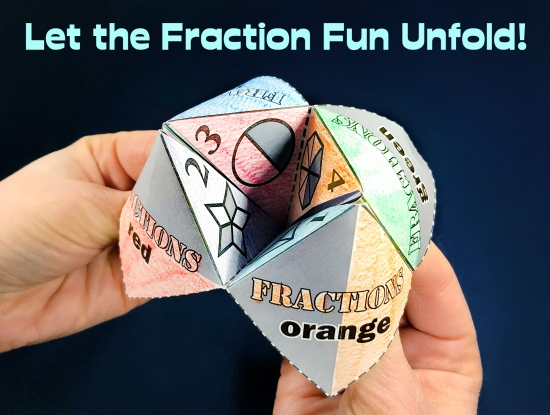# Fun Fraction ResourcesCheck out the Super Teacher Worksheets collection of fraction activities for kids! You'll find a wide variety of resources for fractions topics, including reciprocal fractions, mixed numbers and improper fractions, fractions of sets, comparing and ordering fractions, equivalent fractions and simplifying, basic fractions, adding fractions, subtracting fractions, multiplying fractions, dividing fractions, and more!